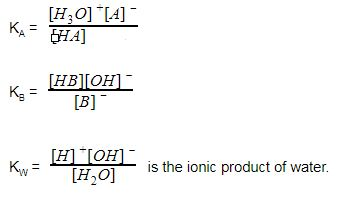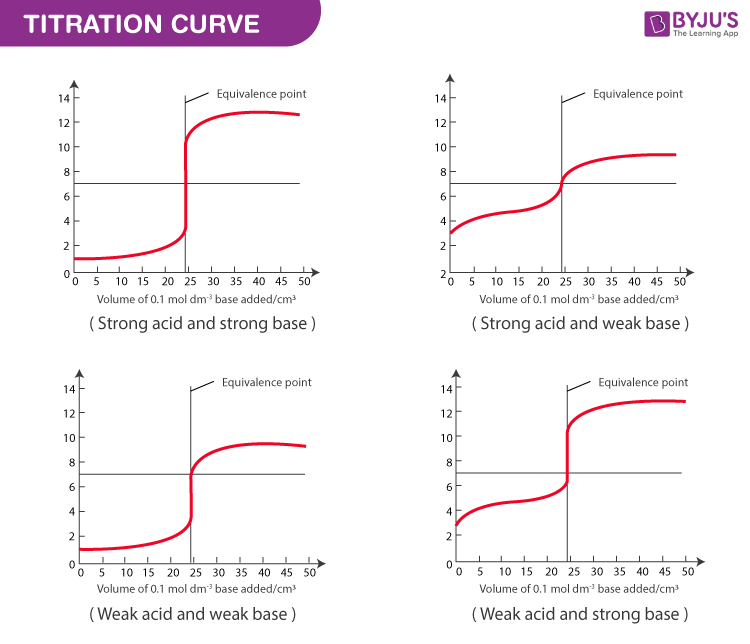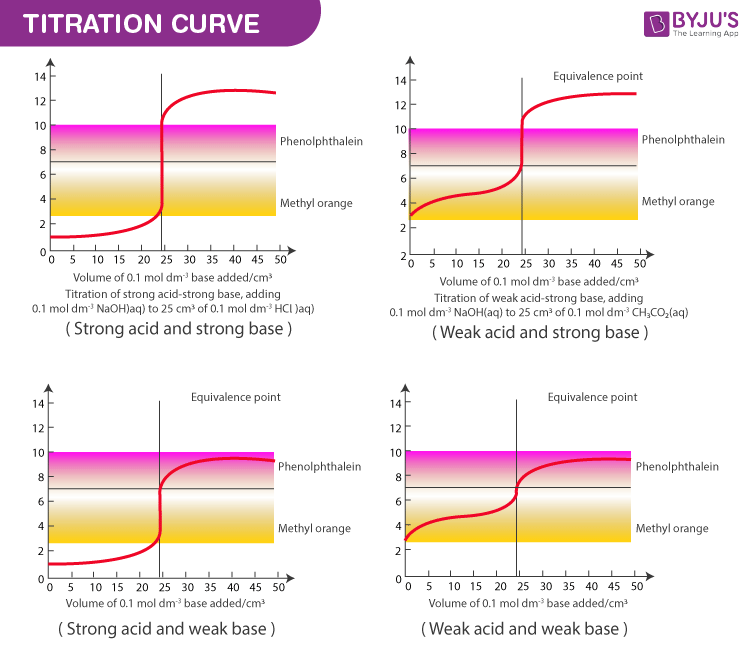# Acid Base Titration

An acid–base titration is a method of quantitative analysis for determining the concentration of an acid or base by exactly neutralizing it with a standard solution of base or acid having known concentration.

## What is Acid-Base Titration?

An acid-base titration is an experimental technique used to acquire information about a solution containing an acid or base.

Hundreds of compounds both organic and inorganic can be determined by a titration based on their acidic or basic properties. Acid is titrated with a base and base is titrated with an acid. The endpoint is usually detected by adding an indicator.

 Also read practise questions: Acid Base Titrations Questions

## Recommended Videos## Learning Objective

To determine the concentration of an acid or base by measuring the volume of titrant (of known concentration) that reacts with it according to a stoichiometric proton-transfer reaction.

## Theory

An acid-base titration involves strong or weak acids or bases. Specifically, an acid-base titration can be used to figure out the following.

1. The concentration of an acid or base
2. Whether an unknown acid or base is strong or weak.
3. pKa of an unknown acid or pKb of the unknown base.

Let us consider acid-base reaction which is proceeding with a proton acceptor. In water, the proton is usually solvated as H3O+. H2O is added to the base to lose (OH) or gain (H3O+). Acid-base reactions are reversible.

The reactions are shown below.

HA + H2O → H3O+ + A(acid)

B + H2O → BH + OH (base)

Here [A] is the conjugate base, B-H is conjugate acid. Thus we say

Acid + Base ⇋ Conjugate base + Conjugate acid

HenceIt is possible to give an expression for [H+] in terms of KA, KB and Kw for a combination of various types of strong and weak acids or bases.

## Key Terms

1. Titration – A process where a solution of known strength is added to a certain volume of a treated sample containing an indicator.
2. Titrant – A solution of known strength of concentration used in the titration.
3. Titrand – The titrand is any solution to which the titrant is added and which contains the ion or species being determined.
4. Titration curve – A plot of pH Vs millilitres of titrant showing the manner in which pH changes Vs millilitres of titrant during an acid-base titration.
5. Equivalence point – The point at which just an adequate reagent is added to react completely with a substance.
6. Buffer solution – A solution that resists changes in pH even when a strong acid or base is added or when it is diluted with water

## Types of Acid-Base Titration

The types and examples of strong/weak acids and bases are tabulated below.

 S.No Types Examples 1. Strong acid-strong base Hydrochloric acid and sodium hydroxide 2. Weak acid-strong base Ethanoic acid and sodium hydroxide 3. Strong acid-weak base Hydrochloric acid and ammonia 4. Weak acid-weak base Ethanoic and ammonia

## Titration Curve & Equivalence Point

In a titration, the equivalence point is the point at which exactly the same number of moles of hydroxide ions have been added as there are moles of hydrogen ions. In a titration, if the base is added from the burette and the acid has been accurately measured into a flask. The shape of each titration curve is typical for the type of acid-base titration.The pH does not change in a regular manner as the acid is added. Each curve has horizontal sections where a lot of bases can be added without changing the pH much. There is also a very steep portion of each curve except for weak acid and the weak base where a single drop of base changes the pH by several units. There is a large change of pH at the equivalence point even though this is not centred on pH 7. This is relevant to the choice of indicators for each type of titration.

## Choice of Indicators

Acid-base indicators are substances which change colour or develop turbidity at a certain pH. They locate equivalence point and also measure pH. They are themselves acids or bases are soluble, stable and show strong colour changes. They are organic in nature.

A resonance of electron isomerism is responsible for colour change. Various indicators have different ionization constants and therefore they show a change in colour at different pH intervals.

Acid-base indicators can be broadly classified into three groups.

• The phthaleins and sulphophthaleins (eg; Phenolphthalein)
• Azo indicators (eg; Methyl orange)
• Triphenylmethane indicators (eg; Malachite green)

The two common indicators used in acid-base titration is Phenolphthalein and methyl orange. In the four types of acid-base titrations, the base is being added to the acid in each case. A graph is shown below where pH against the volume of base added is considered. The pH range over which the two indicators change colour. The indicator must change within the vertical portion of the pH curve.pH Range Over which the Two Indicators Change Colour

The Choice of indicators based on the type of titration is tabulated below.

 Types of titration Indicators Strong acid-strong base Phenolphthalein is usually preferred because of its more easily seen colour change. Weak acid-strong base Phenolphthalein is used and changes sharply at the equivalence point and would be a good choice. Strong acid-weak base Methyl orange will change sharply at the equivalence point. Weak acid-weak base Neither phenolphthalein, nor methyl orange is suitable. No indicator is suitable because it requires a vertical portion of the curve over two pH units.

## What is an Indicator? What Are Acid-Base Indicators?## Solved Example

### Problem:

A 1.2gm sample of a mixture of (Na2CO3 + NaHCO3) is dissolved and titrated with 0.5N HCl. With phenolphthalein, the endpoint is at 15ml while after further addition of methyl orange a second endpoint is at 22ml. Calculate the percentage composition of the mixture.

### Solution:

15 + 15 = 30ml acid is necessary to neutralize Na2CO3 completely.

Total volume needed = 15 + 22 = 37ml

(37-30) = 7ml acid is needed for neutralizing NaHCO3

Therefore, Na2CO3 composition (%) is

= [(30 x 0.5 x 0.053)/1.2] x 100 = 66.25%

= (7 x 0.5 x 0.042 x 100) / 1.2 = 24.50% NaHCO3

## Frequently Asked Questions – FAQs

Q1

### Why is acid base titration important?

The purpose of a strong acid-strong base titration is to determine the acid solution concentration by titrating it with a basic solution of known concentration, or vice versa until there is neutralization. The reaction between a strong acid-base and a strong base will, therefore, result in water and salt.

Q2

### What are the applications of acid-base titration?

An acid-base titration is used to determine the unknown acid or base concentration by neutralizing it with an acid or a known concentration basis. The unknown concentration can be calculated using the stoichiometry of the reaction.

Q3

### Which indicator is used in acid-base titration?

Using a phenolphthalein indicator, a strong acid- strong base titration is performed. Phenolphthalein is selected because it changes colour between 8.3-10 in a pH range. In basic solutions, it will appear pink, and clear in acidic solutions.

Q4

### What are two acid-base indicators?

Examples of acid-base indicators include red cabbage juice, litmus paper, phenolphthalein and. An acid-base indicator is a weak acid or weak base which dissociates in water to produce the weak acid and its conjugate base, or the weak base and its conjugate acid. The species and their conjugate are of different colours.

Q5

### Define the Equivalence point.

The point at which just an adequate reagent is added to react completely with a substance.

Test your knowledge on acid base titration!